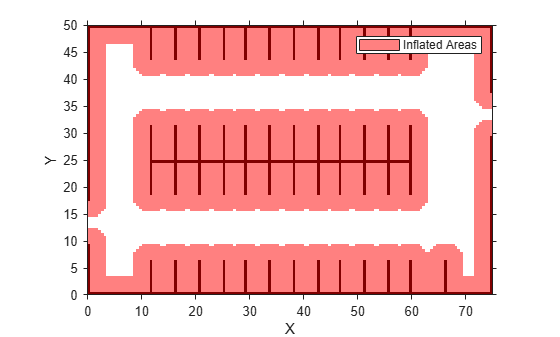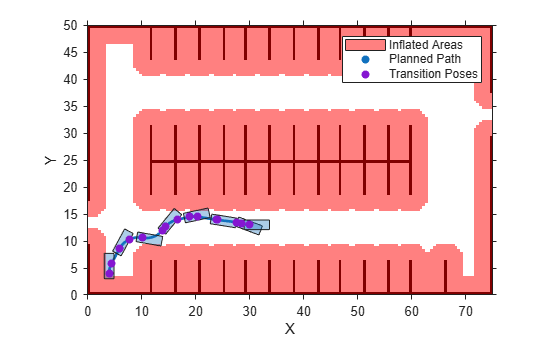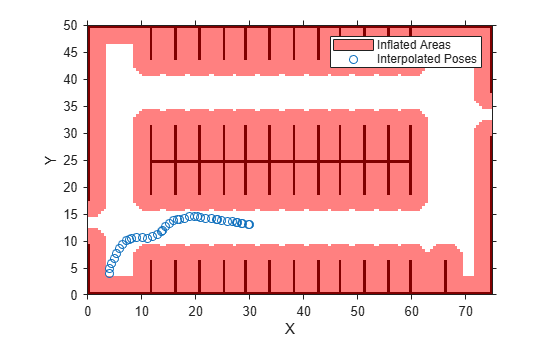# driving.Path

Planned vehicle path

## Description

The `driving.Path` object represents a vehicle path composed of a sequence of path segments. These segments can be either `driving.DubinsPathSegment` objects or `driving.ReedsSheppPathSegment` objects and are stored in the `PathSegments` property of `driving.Path`.

To check the validity of the path against a `vehicleCostmap` object, use the `checkPathValidity` function. To interpolate poses along the length of the path, use the `interpolate` function.

## Creation

To create a `driving.Path` object, use the `plan` function, specifying a `pathPlannerRRT` object as input.

## Properties

expand all

Initial pose of the vehicle, specified as an [x, y, Θ] vector. x and y are in world units, such as meters. Θ is in degrees.

Goal pose of the vehicle, specified as an [x, y, Θ] vector. x and y are in world units, such as meters. Θ is in degrees.

Segments along the path, specified as an array of `driving.DubinsPathSegment` objects or `driving.ReedsSheppPathSegment` objects.

Length of the path, in world units, specified as a positive real scalar.

## Object Functions

 `interpolate` Interpolate poses along planned vehicle path `plot` Plot planned vehicle path

## Examples

collapse all

Plan a vehicle path through a parking lot by using the optimal rapidly exploring random tree (RRT*) algorithm. Check that the path is valid, and then plot the transition poses along the path.

Load a costmap of a parking lot. Plot the costmap to see the parking lot and inflated areas for the vehicle to avoid.

```data = load('parkingLotCostmap.mat'); costmap = data.parkingLotCostmap; plot(costmap)```Define start and goal poses for the vehicle as [x, y, Θ] vectors. World units for the (x,y) locations are in meters. World units for the Θ orientation angles are in degrees.

```startPose = [4, 4, 90]; % [meters, meters, degrees] goalPose = [30, 13, 0];```

Use a `pathPlannerRRT` object to plan a path from the start pose to the goal pose.

```planner = pathPlannerRRT(costmap); refPath = plan(planner,startPose,goalPose);```

Check that the path is valid.

`isPathValid = checkPathValidity(refPath,costmap)`
```isPathValid = logical 1 ```

Interpolate the transition poses along the path.

`transitionPoses = interpolate(refPath);`

Plot the planned path and the transition poses on the costmap.

```hold on plot(refPath,'DisplayName','Planned Path') scatter(transitionPoses(:,1),transitionPoses(:,2),[],'filled', ... 'DisplayName','Transition Poses') hold off```Plan a vehicle path through a parking lot by using the rapidly exploring random tree (RRT*) algorithm. Interpolate the poses of the vehicle at points along the path.

Load a costmap of a parking lot. Plot the costmap to see the parking lot and inflated areas for the vehicle to avoid.

```data = load('parkingLotCostmap.mat'); costmap = data.parkingLotCostmap; plot(costmap)```Define start and goal poses for the vehicle as `[`x, y, Θ`]` vectors. World units for the (x,y) locations are in meters. World units for the Θ orientation angles are in degrees.

```startPose = [4, 4, 90]; % [meters, meters, degrees] goalPose = [30, 13, 0]; ```

Use a `pathPlannerRRT` object to plan a path from the start pose to the goal pose.

```planner = pathPlannerRRT(costmap); refPath = plan(planner,startPose,goalPose);```

Interpolate the vehicle poses every 1 meter along the entire path.

```lengths = 0 : 1 : refPath.Length; poses = interpolate(refPath,lengths);```

Plot the interpolated poses on the costmap.

```plot(costmap) hold on scatter(poses(:,1),poses(:,2),'DisplayName','Interpolated Poses') hold off```## Compatibility Considerations

expand all

Not recommended starting in R2018b

## Extended Capabilities

### C/C++ Code GenerationGenerate C and C++ code using MATLAB® Coder™.

Introduced in R2018a